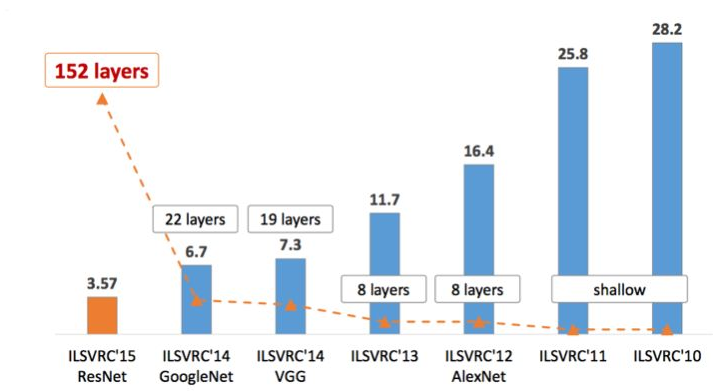# 背景¶

ResNet这个里程碑式的创新对AI领域带来了深远的影响。然而，作者发现ResNet网络中不是所有的层都是必要的，因此结合经典的Dropout思想提出在训练过程中随机丢弃丢掉一些层来优化ResNet的训练过程。(PS：这不就是把Dropout用到网络层这个单位吗？)

# 结构¶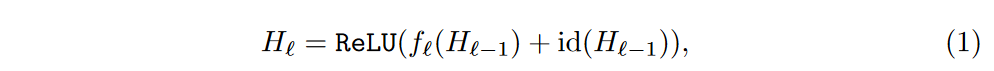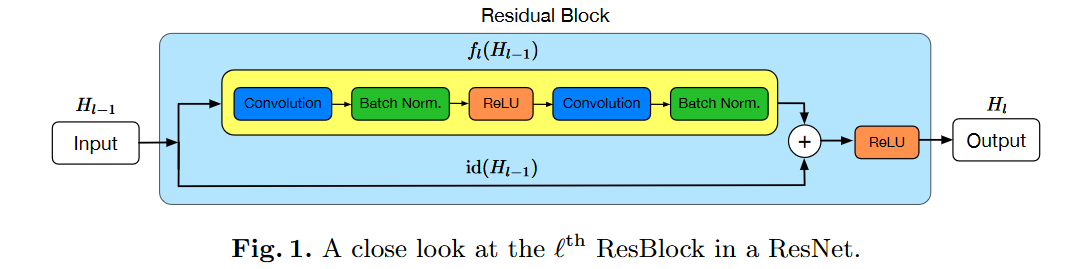Stochastic Depth(随机深度网络)就是在训练时加入了一个随机变量$b$，其中$b$的概率分布是满足一个伯努利分布的，然后将$f$乘以$b$，对残差部分做了随机丢弃。如果$b=1$，这个结构即是原始的ResNet结构，而当$b=0$时，残差支路没有被激活，整个结构退化为一个恒等函数。这个过程可以用下面的等式来表示：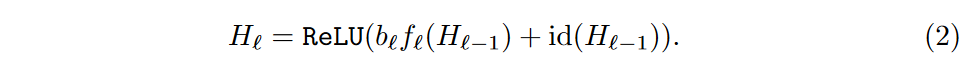• 一种设置方式是将其设置为同一个值，即$p_l=p$，其中$p_l$代表每个残差块$l$$p$参数取值。
• 另外一种设置方式是将其设置为残差层数$l$的平滑函数。从$p_0=1$线性递减到$p_L=0.5$，一共有L个残差块。公式表示如下：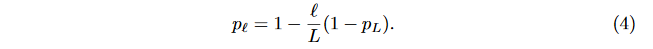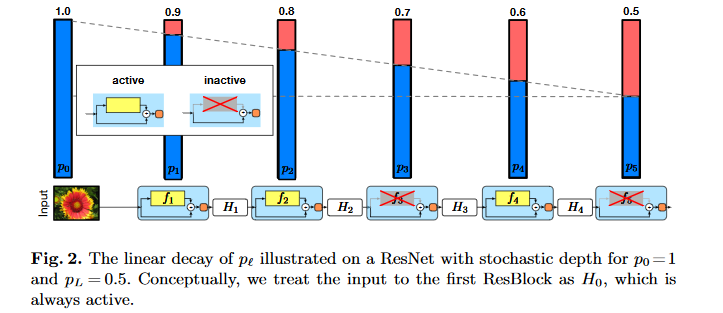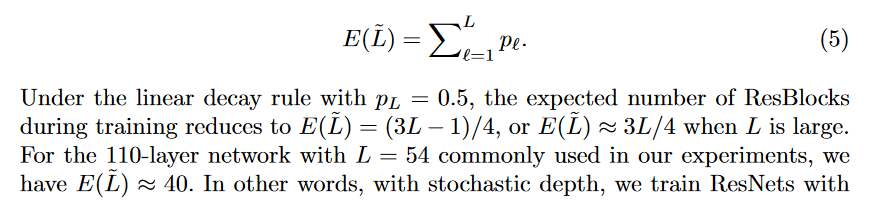# 测试¶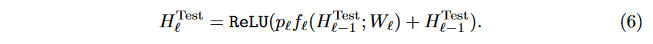# 实验¶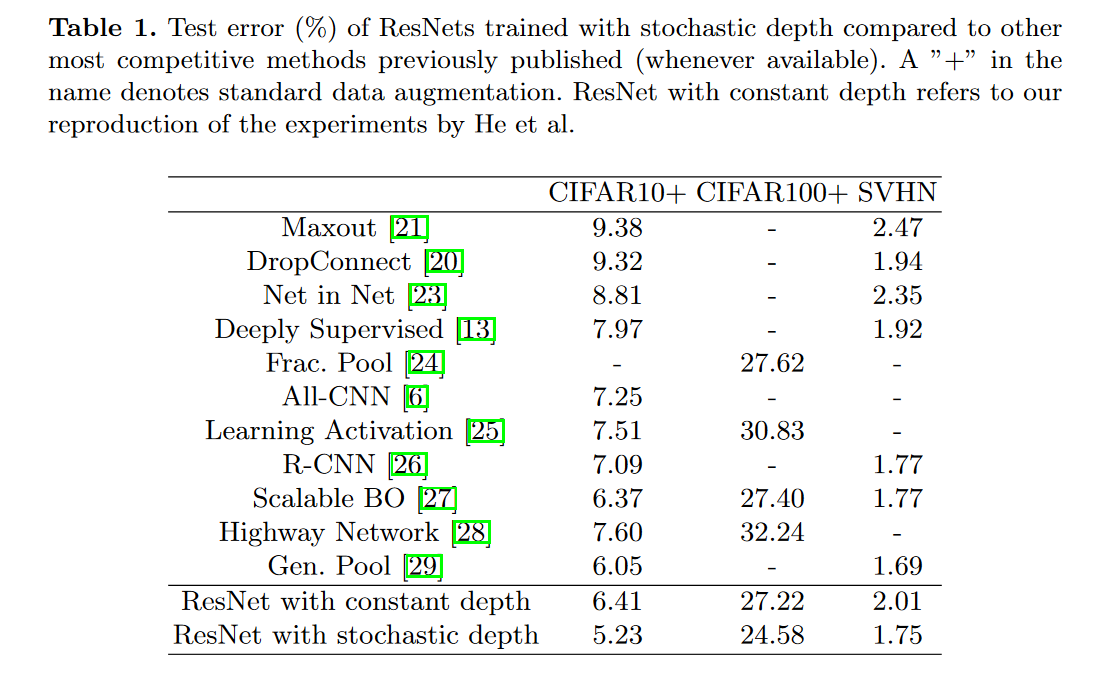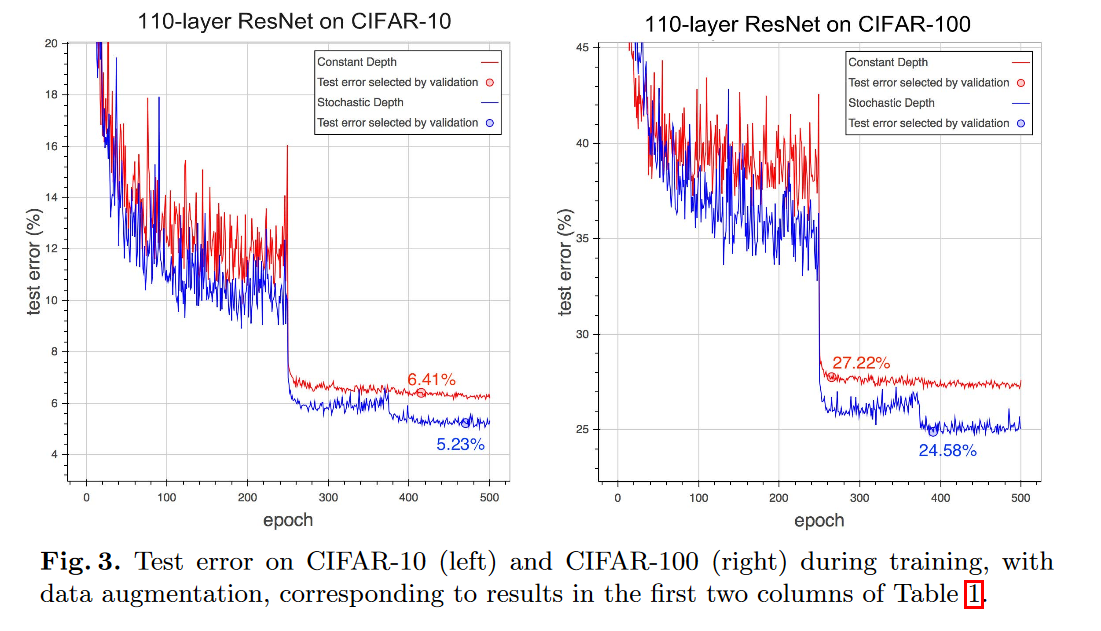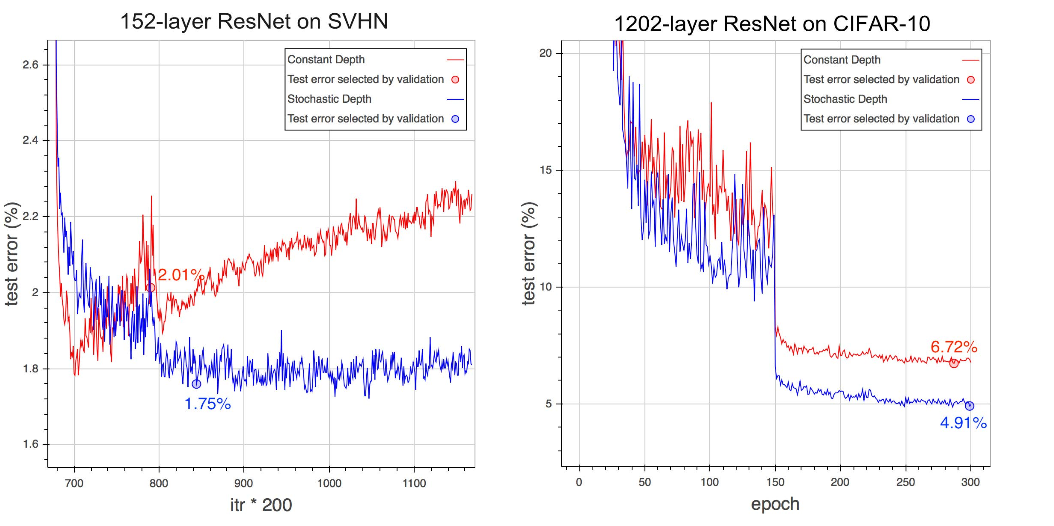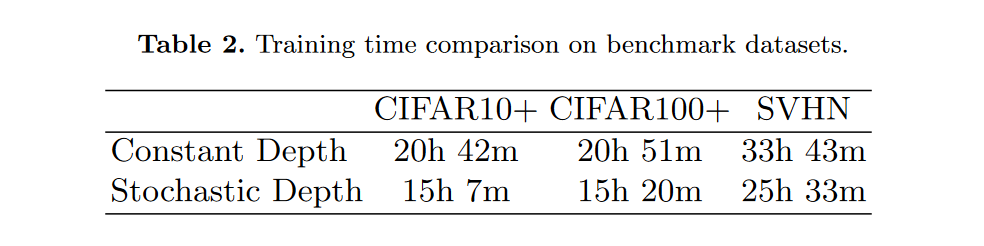# 为什么随机深度网络有效？¶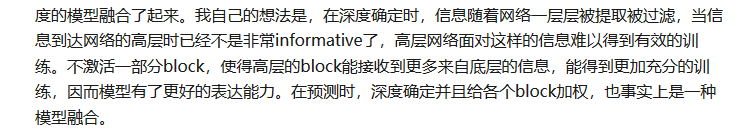# 代码实现¶

def residual_drop(x, input_shape, output_shape, strides=(1, 1)):

nb_filter = output_shape
conv = Convolution2D(nb_filter, 3, 3, subsample=strides,
border_mode="same", W_regularizer=l2(weight_decay))(x)
conv = BatchNormalization(axis=1)(conv)
conv = Activation("relu")(conv)
conv = Convolution2D(nb_filter, 3, 3,
border_mode="same", W_regularizer=l2(weight_decay))(conv)
conv = BatchNormalization(axis=1)(conv)

if strides >= 2:
x = AveragePooling2D(strides)(x)

if (output_shape - input_shape) > 0:
output_shape - input_shape,
output_shape,
output_shape)
x = Lambda(lambda y: K.concatenate([y, padding], axis=1),
output_shape=output_shape)(x)

_death_rate = K.variable(death_rate)
scale = K.ones_like(conv) - _death_rate
conv = Lambda(lambda c: K.in_test_phase(scale * c, c),
output_shape=output_shape)(conv)

out = merge([conv, x], mode="sum")
out = Activation("relu")(out)

gate = K.variable(1, dtype="uint8")
add_tables += [{"death_rate": _death_rate, "gate": gate}]
return Lambda(lambda tensors: K.switch(gate, tensors, tensors),
output_shape=output_shape)([out, x])


# 卷积神经网络学习路线往期文章¶